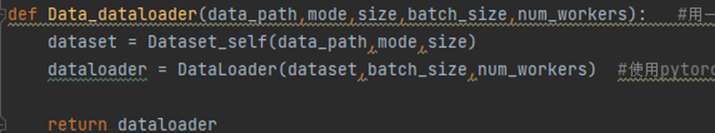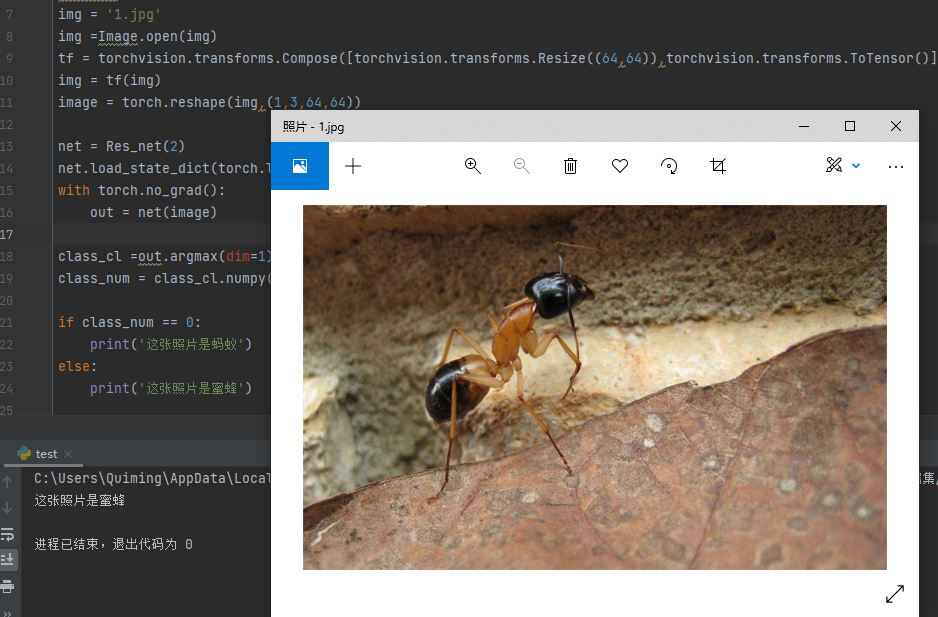﻿ 聊聊基于pytorch实现Resnet对本地数据集的训练问题_python_脚本之家
python# 聊聊基于pytorch实现Resnet对本地数据集的训练问题

mian.py文件是该项目的总文件，也是训练网络模型的运行文件，文本的介绍流程是随着该文件一 一对代码进行介绍。

main.py代码如下所示：

```from dataset import data_dataloader    #电脑本地写的读取数据的函数
from torch import nn                   #导入pytorch的nn模块
from torch import optim                #导入pytorch的optim模块
from network import Res_net            #电脑本地写的网络框架的函数
from train import train                #电脑本地写的训练函数

def main():
# 以下是超参数的定义
lr = 1e-4           #学习率
epochs = 10         #训练轮次
model = Res_net(2)  # resnet网络
optimizer = optim.Adam(model.parameters(), lr=lr)  # 优化器
loss_function = nn.CrossEntropyLoss()  # 损失函数
# 训练以及验证测试函数
if __name__ == '__main__':
main()```

main.py流程图如图1所示：### 1.dataset.py（先看代码的总体流程再看介绍）

dataset.py文件代码如下所示：

```import torch
import os,glob
import random
import csv
from torch.utils.data import Dataset
from PIL import Image
from torchvision import transforms

# 第一部分：通过三个步骤得到输出的tensor类型的数据
class Dataset_self(Dataset):                    #如果是nn.moduel 则是编写网络模型框架，这里需要继承的是dataset的数据，所以括号中的是Dataset
#第一步：初始化
def __init__(self,root,mode,resize,):       #root是文件根目录，mode是选择什么样的数据集，resize是图像重新调整大小
super(Dataset_self, self).__init__()
self.resize = resize
self.root = root
self.name_label = {}       #创建一个字典来保存每个文件的标签
#首先得到标签相对于的字典（标签和名称一一对应）
for name in sorted(os.listdir(os.path.join(root))):     #排序并且用列表的形式打开文件夹
if not os.path.isdir(os.path.join(root,name)):      #不是文件夹就不需要读取
continue
self.name_label[name] = len(self.name_label.keys())  #每个文件的名字为name_Label字典中有多少对键值对的个数
#print(self.name_label)
self.image,self.label = self.make_csv('images.csv')       #编写一共函数来读取图片和标签的路径
#在得到image和label的基础上对图片数据进行一共划分  （注意：如果需要交叉验证就不需要验证集，只划分为训练集和测试集）
if mode == 'train':
self.image ,self.label= self.image[:int(0.6*len(self.image))],self.label[:int(0.6*len(self.label))]
if mode == 'val':
self.image ,self.label= self.image[int(0.6*len(self.image)):int(0.8*len(self.image))],self.label[int(0.6*len(self.label)):int(0.8*len(self.label))]
if mode == 'test':
self.image ,self.label= self.image[int(0.8*len(self.image)):],self.label[int(0.8*len(self.label)):]
# 获得图片和标签的函数
def make_csv(self,filename):
if not os.path.exists(os.path.join(self.root,filename)):  #如果不存在汇总的目录就新建一个
images = []
for image in self.name_label.keys():                            # 让image到name_label中的每个文件中去读取图片
images += glob.glob(os.path.join(self.root,image,'*jpg'))   #加* 贪婪搜索关于jpg的所有文件
#print('长度为：{}，第二张图片为：{}'.format(len(images),images))
random.shuffle(images)                                         #把images列表中的数据洗牌
# images: ./data\ants\382971067_0bfd33afe0.jpg
with open(os.path.join(self.root,filename),mode='w',newline='') as f :  #创建文件
writer = csv.writer(f)
for image in images:
name = image.split(os.sep)[-2]  #得到与图片相对应的标签
label = self.name_label[name]
writer.writerow([image,label])  #写入文件  第一行：./data\ants\382971067_0bfd33afe0.jpg,0
images,labels = [],[]
with open(os.path.join(self.root,filename)) as f:   #读取文件
image, label = row
label = int(label)
images.append(image)
labels.append(label)
assert len(images) == len(labels)   #类似if语句，只有两者长度一致才继续执行，否则报错
return images,labels                #返回所有！！是所有的图片和标签（此处的图片不是图片数据本身，而是它的文件目录）
#第二步：得到图片数据的长度（标签数据长度与图片一致）
def __len__(self):
return len(self.image)
#第三步：读取图片和标签，并输出
def __getitem__(self, item):   # 单张返回张量的图像与标签
image,label = self.image[item],self.label[item]      #得到单张图片和相应的标签（此处都是image都是文件目录）
image = Image.open(image).convert('RGB')             #得到图片数据
#使用transform对图片进行处理以及变成tensor类型数据
transf = transforms.Compose([transforms.Resize((int(self.resize),int(self.resize))),
transforms.RandomRotation(15),
transforms.CenterCrop(self.resize),
transforms.ToTensor(),  #先变成tensor类型数据，然后在进行下面的标准化
])
image = transf(image)
label = torch.tensor(label)   #把图片标签也变成tensor类型
return image,label
dataset = Dataset_self(data_path,mode,size)
#测试
def main():
test = Dataset_self('./data','train',64)
if __name__ == '__main__':
main()```

dataset.py流程图2所示：make_csv函数中，首先判断是否以及存在我们需要的文件，如果存在则直接读取，如果不存在就先生成一个存储所有图片目录和标签的文件。Dataset部分其功能简单概括就是将本地数据集中的图片和标签变成tensor类型数据读取为需要使用的数据集。

### 2.network.py

main.py()中，我们定义了一些超参数等，分别有学习率，训练轮次，训练模型，优化器以及损失函数。对于训练模型，本文使用的是本地编写的一个小型的Resnet模型。其代码如下所示：

```import torch
from torch import nn

# 先写好resnet的block块
class Res_block(nn.Module):
def __init__(self,in_num,out_num,stride):
super(Res_block, self).__init__()
self.bn1 = nn.BatchNorm2d(out_num)
self.bn2 = nn.BatchNorm2d(out_num)
self.extra = nn.Sequential(
nn.Conv2d(in_num,out_num,(1,1),stride=stride),
nn.BatchNorm2d(out_num)
)   #使得输入前后的图像数据大小是一致的
self.relu = nn.ReLU()
def forward(self,x):
out = self.relu(self.bn1(self.cov1(x)))
out = self.relu(self.bn2(self.cov2(out)))
out = self.extra(x) + out
return out
class Res_net(nn.Module):
def __init__(self,num_class):
super(Res_net, self).__init__()
self.init = nn.Sequential(
nn.Conv2d(3,16,(3,3)),
nn.BatchNorm2d(16)
)   #预处理
self.bn1 = Res_block(16,32,2)
self.bn2 = Res_block(32,64,2)
self.bn3 = Res_block(64,128,2)
self.bn4 = Res_block(128,256,2)
self.fl = nn.Flatten()
self.linear1 = nn.Linear(8192,10)
self.linear2 = nn.Linear(10,num_class)
out = self.relu(self.init(x))
#print('inint:',out.shape)
out = self.bn1(out)
#print('bn1:', out.shape)
out = self.bn2(out)
#print('bn2:', out.shape)
out = self.bn3(out)
#print('bn3:', out.shape)
out = self.fl(out)
#print('flatten:', out.shape)
out = self.relu(self.linear1(out))
#print('linear1:', out.shape)
out = self.relu(self.linear2(out))
#print('linear2:', out.shape)
#测试
def main():
x = torch.randn(2,3,64,64)
net = Res_net(2)
out = net(x)
print(out.shape)
if __name__ == '__main__':
main()```

network.py流程图如图10所示：Resnet模型网络主要是两部分，首先编写resnet中的每个残差块，然后编写整个网络。在开始介绍代码之前，首先用我的理解来介绍一下Resnet，也就是残差网络的思想与逻辑（具体可以搜索其他资料查看）。残差网络其主要的目的是能够训练一个深层次的网络，希望是随着网络的加深，效果越来越好。但是因为网络加深，很有可能一些参数会得不到训练（一次次的迭代，使得梯度消失），所有Resnet网络巧妙的运用了一个残差块来解决因为网络模型太深而导致其梯度消失的问题，如图11所示：### 3.train.py

train.py是整个模型的训练过程，本文将其打包成为一个函数，然后在mian.py中调用，因为基本上网络的训练过程都大同小异，一般都是用训练集训练，在验证集上得到最好的轮次，最后保存网络参数并且在测试集上检测，所以这里直接将训练过程和验证过程打包成为函数，便于以后项目的直接调用。

train.py代码如下所示：

```import torch
from torch import optim
from dataset import Dataset_self
from network import Res_net
from torch import nn
from matplotlib import pyplot as plt
import numpy as np

correct = 0
logits = model(x)
pred = logits.argmax(dim=1)     #得到logits中分类值（要么是[1,0]要么是[0,1]表示分成两个类别）
correct += torch.eq(pred,y).sum().float().item()        #用logits和标签label想比较得到分类正确的个数
return correct/total
#把训练的过程定义为一个函数
def train(model,optimizer,loss_function,train_data,val_data,test_data,epochs):  #输入：网络架构，优化器，损失函数，训练集，验证集，测试集，轮次
best_acc,best_epoch =0,0      #输出验证集中准确率最高的轮次和准确率
train_list,val_List = [],[]   # 创建列表保存每一次的acc，用来最后的画图
for epoch in range(epochs):
print('============第{}轮============'.format(epoch + 1))
for steps,(x,y) in enumerate(train_data):   #  for x,y in train_data
logits = model(x)                   #数据放入网络中
loss = loss_function(logits,y)      #得到损失值
loss.backward()                     #后向传播
optimizer.step()
train_acc =evaluate(model,train_data)
train_list.append(train_acc)
print('train_acc',train_acc)
#if epoch % 1 == 2:   #这里可以设置每两次训练验证一次
val_acc = evaluate(model,val_data)
print('val_acc=',val_acc)
val_List.append((val_acc))
if val_acc > best_acc:  #判断每次在验证集上的准确率是否为最大
best_epoch = epoch
best_acc = val_acc
torch.save(model.state_dict(),'best.mdl')   #保存验证集上最大的准确率
print('===========================分割线===========================')
print('best acc:',best_acc,'best_epoch:',best_epoch)
#在测试集上检测训练好后模型的准确率
print('detect the test data!')
test_acc = evaluate(model,test_data)
print('test_acc:',test_acc)
train_list_file = np.array(train_list)
np.save('train_list.npy',train_list_file)
val_list_file = np.array(val_List)
np.save('val_list.npy',val_list_file)
#画图
x_label = range(1,len(val_List)+1)
plt.plot(x_label,train_list,'bo',label='train acc')
plt.plot(x_label,val_List,'b',label='validation acc')
plt.title('train and validation accuracy')
plt.xlabel('epochs')
plt.legend()
plt.show()
#测试
def main():
train_dataset = Dataset_self('./data', 'train', 64)
vali_dataset = Dataset_self('./data', 'val', 64)
test_dataset = Dataset_self('./data', 'test', 64)
lr = 1e-4
epochs = 5
model = Res_net(2)
criteon = nn.CrossEntropyLoss()
train(model,optimizer,criteon,train_loaber,val_loaber,test_loaber,epochs)
if __name__ == '__main__':
main()```

train.py流程图如图15所示：train函数内部首先定义验证集中最好的准确率和最好的轮次，然后创建两个列表来保存每一次的训练集和验证集的准确率（用来画图查看），然后就是进行epochs次训练。### 4.结果与总结```from network import Res_net
import torch
from PIL import Image
import torchvision

#导入图片
img = '1.jpg'
img =Image.open(img)
tf = torchvision.transforms.Compose([torchvision.transforms.Resize((64,64)),torchvision.transforms.ToTensor()])
img = tf(img)
image = torch.reshape(img,(1,3,64,64))
#加载模型
net = Res_net(2)
out = net(image)
#确定分类
class_cl =out.argmax(dim=1)
class_num = class_cl.numpy()
if class_num == 0:
print('这张照片是蚂蚁')
else:
print('这张照片是蜜蜂')```